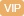| APP | 校园号 | 客服 客服热线：400-863-9889

400-863-9889（2017秋•重庆期末）用竖式计算．
 56×8= 504×4= 180×5= 75÷3= 68÷7= 82÷6=
【考点】整数的乘法及应用整数的除法及应用【专题】计算题．
（1）根据等式的性质，方边同减27求解；

 5 4
x，再依据等式的性质，方程两同以
 5 4

12根式的性质，在方程同时减2.7，再同时除以6求解；

（）根据等式性质，在方程两边同时乘1.8；

（7）依据的性质，方两同时除以7求解；
（3）先化等式的左边为
 7 9
x，再依据的质，再除以
 7 9

【解答】解：7X2=13.5，
=315；
27+X-2=10-2，
1.1X1.15=
 1 20
1.15，
6X+4x=1.28-4X4x，
（）X-2.5=0.，
25x1，

 5 4
X=4
 1 4

X=
 1 23

X=4；
2.=10，
9.6X17.28，
6X÷67.5，

 7 9
X=2.1，

 5 4
X÷
 5 4
=4
 1 4
÷
 5 4

 7 9
X÷
 7 9
=3.1÷
 7 9

2.X÷2.5=1÷2.，
77=38.5÷7，
（1-7.5X=0.125×，
X=37.8；
0.6X+-2=21-22，
13.3；
7X÷7=847，
860%X+22=21，
X=0.4；
6+2.-2.7=102-2.7，
.6=17.28-4，
0.X÷0.6=189÷0.6，
0.6X=89，
X=1.25；
-2.5+2.5.8+2.5，
（10）+X=103，
1）6X+.7=10.2，
（X-.2）=.5X+5.2，
9.6÷9.=17.89.6，
=76；
（11）X÷1.82，
7385，
.15X=
 1 20

X=29.7．
【点评】本题方程主要用了等性即“等式两边时同一个数，或以同一个不为0的，果仍相”，“等式两边同时加减）同一个（或式子），结果仍相等”．解方时注意对齐号．

0/0